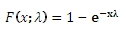# SQL Server EXPON.INV function

EXPINV

Updated: 31 July 2010

Use EXPINV to calculate the inverse of the cumulative distribution function of the exponential distribution.

The equation for the cumulative distribution function is:Syntax
SELECT [wctStatistics].[wct].[EXPINV] (
<@CDF, float,>
,<@Lambda, float,>)
Arguments
@CDF
is the cumulative distribution function. @CDF is an expression of type float or of a type that implicitly converts to float
@Lambda
is the parameter value. @Lambda is an expression of type float or of a type that implicitly converts to float
Return Types
float
Remarks
·         @P must be greater than or equal to zero and less than on (0 ≤ @P < 1).
·         @Lambda must be greater than zero (0 < @Lambda).
Examples
Calculate the cumulative distribution function:
SELECT wct.EXPONDIST(0.75, 9.5, 'True')

This produces the following result
----------------------
0.999195266989875

(1 row(s) affected)

Calculate the inverse:
SELECT dbo.EXPINV(0.999195266989875,9.5)

This produces the following result
----------------------
0.749999999999955

(1 row(s) affected)

### SupportCopyright 2008-2021 Westclintech LLC         Privacy Policy        Terms of Service